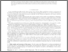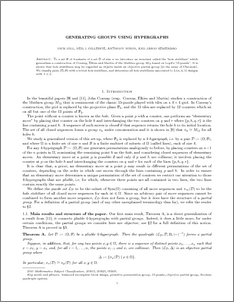# Generating groups using hypergraphs

Gill, Nick and Gillespie, Neil and Nixon, Anthony Keith and Semeraro, Jason (2016) Generating groups using hypergraphs. The Quarterly Journal of Mathematics, 67 (1). pp. 29-52. ISSN 0033-5606Preview
PDF (gen)
gen.pdf - Accepted Version
To a set $\B$ of 4-subsets of a set $\Omega$ of size $n$ we introduce an invariant called the hole stabilizer' which generalises a construction of Conway, Elkies and Martin of the Mathieu group $M_{12}$ based on Loyd's 15-puzzle'. It is shown that hole stabilizers may be regarded as objects inside an objective partial group (in the sense of Chermak). We classify pairs $(\Omega,\B)$ with a trivial hole stabilizer, and determine all hole stabilizers associated to $2$-$(n,4,\lambda)$ designs with $\lambda \leq 2$.View Item